## Graphics Gallery

#### Some mathematical images by Adam Coffman, rendered using POV-Ray v3.1 or v3.6, or Maple.

 Click on the small picture to see the larger picture. Click on the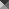square to load an animated gif.An article of K. Boyadzhiev in the September 2007 issue of The College Mathematics Journal proposes a notion of an "Equiangular surface." The picture links to a page showing two pictures of an example from that paper.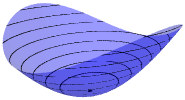The picture links to a page about surfaces which are graphs of functions with critical points. This is also related to my Memoir, Unfolding CR Singularities.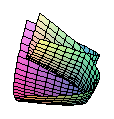The picture links to a page about affine surfaces parametrized by quadratic polynomials.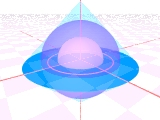The picture illustrates some of the domains that appear in a joint paper on A Taylor series condition for harmonic extension.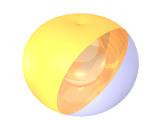The picture links to a page about the hippopede of Proclus and some related special plane curves.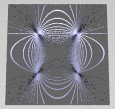The picture links to a page about linear systems of conics.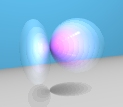The picture links to a page about linear systems of ellipsoids. Here's a page of photos and lecture notes from a math club talk I gave about ellipsoid packing.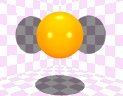A deflating ellipsoid. The animation demonstrates the ellipsoids from Examples 4.6 and 4.7 of my paper on CR singular immersions of complex projective spaces. The two points on the ellipsoid that don't move during the animation, (0,0,1) and (0,0,-1), have tangent planes parallel to the xy-plane (the wall on the left). The "Bishop invariant" at these points starts with value 0, when the surface is a sphere, and increases, with upper bound 1/2, until the surface flattens into an elliptical disc in the yz-plane. This animation is also an example of a deformation of an elliptic point, as described in my paper on Unfolding CR singularities. Yet another phenomenon demonstrated by this sequence is how a Steiner surface can collapse into two dimensions. At t = 0 (the first frame of the animation, shown in the still picture), these parametric equations (below) define a sphere. For 0 < t < 1, the image is an ellipsoid. As t increases to 1, the parametric component 2(1-t)u0u1 goes to zero, so at t = 1, the image is contained in the yz-plane. The implicit equation changes from an ellipsoid for t < 1, to the entire yz-plane at t = 1. The animation continues by decreasing the parameter t back to 0, re-inflating the ellipsoid. real quadratic homogeneous parametrization: [u02+u12+u22 : 2(1-t)u0u1 : 2(-1-t)u0u2 : -u02+u12+u22)] affine implicit equation: (1+t)2x2+(1-t)2y2+(1-t2)2z2-(1-t2)2 = 0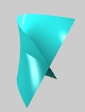This is the Whitney umbrella, a ruled surface. You can see the "double line," where the surface intersects itself, and the "pinch point," where the double line terminates in a singularity. The lines on the surface actually extend indefinitely, so this picture only shows the part of the object inside a bounding box. Using real parameters u, v, the surface can be described by the quadratic parametric equations (x,y,z)=(u,v2,uv), so... The Whitney Umbrella is an example of a Steiner surface (Type 8). It also is a representative from the Peters-Reif classification of quadratically parametrized affine surfaces (Type 3-2-2a). These equations are Whitney's local normal form for generic cross-cap singularities of smooth 2-parameter maps in space. Whitney's 1943 paper in Duke Math. J. Whitney's umbrella can also be described as a cubic variety, since the points (x,y,z) on the surface satisfy the implicit equation x2y - z2 = 0. However, all points of the form (0,y,0) satisfy this equation, so the solution set of the cubic includes the entire double line (the y-axis is the handle of the umbrella), instead of just the closed ray of self-intersection (where u=0 and y=v2 is nonnegative). In the POV code, I used the cubic implicit equation, but clipped off the negative y-axis by the bounding box.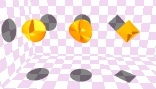This picture shows a Roman surface, together with two objects with a similar shape. It is intended to aid in the visualization of this type of Steiner surface, with its six pinch points, three double lines, and one triple point. The Euclidean volume and surface area of the three objects are also compared, but other Roman surfaces related to this one by a linear transformation will of course have different values for volume and area. The polyhedral shape is called a Reinhardt heptahedron or a tetrahemihexahedron.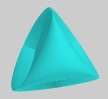More Roman surfaces.The picture links to the "Boy Surface" section on my Steiner surface page. There is a larger picture of the Boy Surface and also an animation showing a deformation of Steiner's Roman Surface into the Boy surface.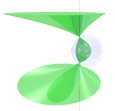The picture links to a page about the surfaces of Gerhard Miehlich.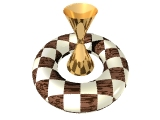This picture shows a hyperboloid and a torus. The interesting parts are the checker pattern (not all squares!) on the torus, and the reflection of the doughnut hole in the hyperboloid.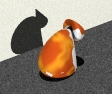The "real algebraic variety" associated to a polynomial p(x,y,z) is the set of all points (x,y,z) that are solutions of the equation p(x,y,z) = 0. A "real analytic" function is defined by an infinite sum of terms, and it can similarly describe a "real analytic variety." Sometimes using finitely many terms in the series for an analytic function, to get a polynomial approximation, is called "chopping off the tail." The picture links to a demonstration of how the solution sets of some equations can look like my cat.

For more equations, images, animations, and links about Steiner surfaces and the Boy surface, see my page on Steiner surfaces.

Back to Adam Coffman's page